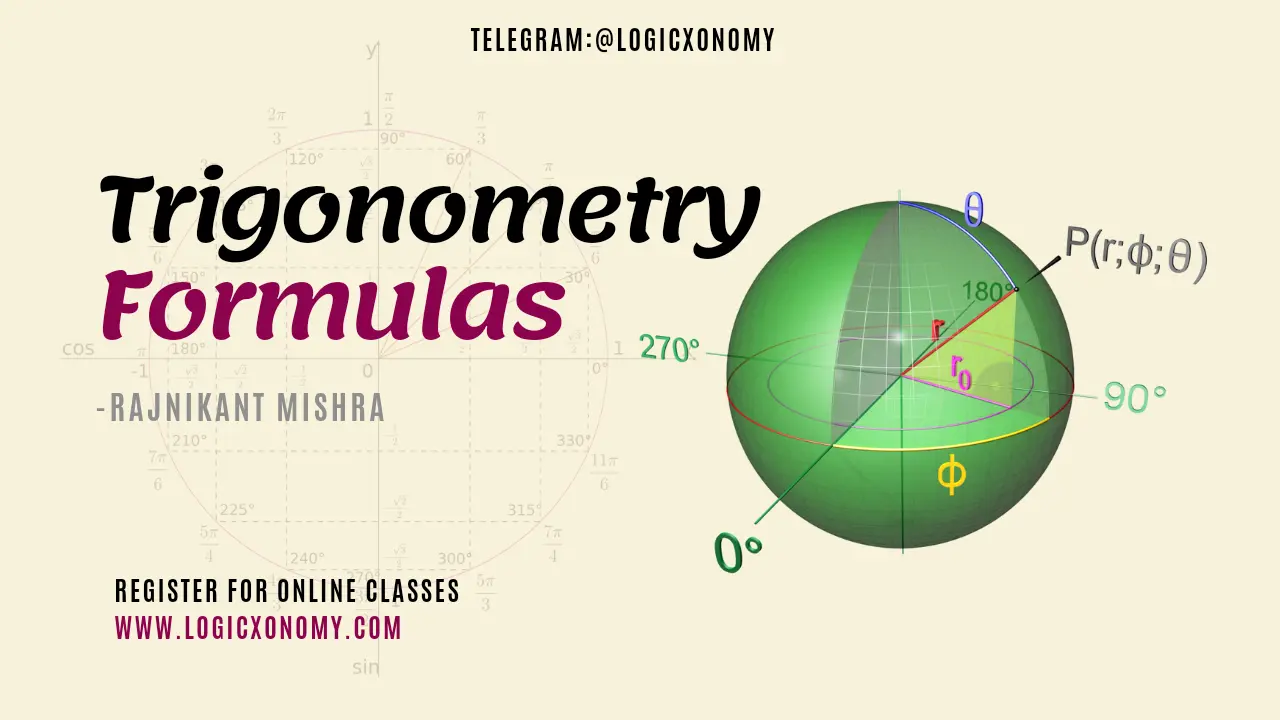Contents

Some very useful Trigonometry formulas and Identities with proof are given here. Here we would also discuss some of the very important practical applications of these formulas in real life with examples.

## Sine Formula

Let, In a △ABC, the length of sides: BC=a, CA=b, and AB=c then according to the Sine Formula

$\frac{a}{Sin(A)}=\frac{b}{Sin(B)}=\frac{c}{Sin(C)}=2R$.

Here R is the Circumradius of the △ABC.

Here is the proof of this formula.

In △BDA, $Sin(B)=\frac{h}{c}$.

h=c.Sin(B)

Similarly, In △CDA

$Sin(C)=\frac{h}{b}$.

h=b.Sin(C)

So, c.Sin(B)=b.Sin(C)

$\frac{b}{Sin(B)}=\frac{c}{Sin(C)}$ ……………(i)

Now, Draw BE⟂AC, the height BE=k

$\frac{a}{Sin(A)}=\frac{c}{Sin(C)}$ ……………(ii)

From the equation (i) and (ii):

$\frac{a}{Sin(A)}=\frac{b}{Sin(b)}=\frac{c}{Sin(C)}$ ……………(iii)

Draw a Circumcircle of △ABC. Here O is the Circumcenter of the triangle.

So, the length of the diameter, BD=2R

∠BAC=∠BDC=∠A (Angles, subtended by the same arc at the circumference in the same segment)

∠BCD=90° (Angle made by the diameter at the Circumference)

Now in △BCD, according to Sine Rule:

$\frac{BC}{Sin(BDC)}=\frac{BD}{Sin(90°)}$.

$\frac{a}{Sin(A)}=\frac{2R}{1}$.

$\frac{a}{Sin(A)}=2R$ …………(iv)

From equation (iii) and (iv):

$\frac{a}{Sin(A)}=\frac{b}{Sin(B)}=\frac{c}{Sin(C)}=2R$

## Cosine Formula

Let, In an △ABC, the length of sides: BC=a, CA=b, and AB=c. BD is perpendicular drawn from vertex B to side AC, here h is the height of the triangle.

Then, According to Cosine Formula:

a2=b2+c2-2bc×Cos(A)

b2=c2+a2-2ac×Cos(B)

c2=a2+b2-2ab×Cos(C)

Here is the proof of this formula.

Apply Pythagoras Theorem in △BDC

a2=h2+DC2

R.H.S.

$Cos(A)=\frac{AD}{AB}=\frac{AD}{c}$.

So, a2=b2+c2-2.bc.Cos(A)

Similarly, we can prove other combinations also.

In △ADB, $Cos(A)=\frac{AD}{c}$.

In △CDB, $Cos(C)=\frac{CD}{a}$.

CD=a.Cos(C) ………(ii)

AC=c.Cos(A)+a.Cos(C)

b=c.Cos(A)+a.Cos(C) ……… (iii)

Similarly, we can prove:

a=b.Cos(C)+c.Cos(B) ……… (iv)

c=a.Cos(B)+b.Cos(A)  ……… (v)